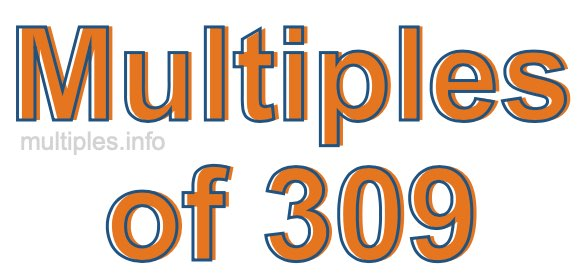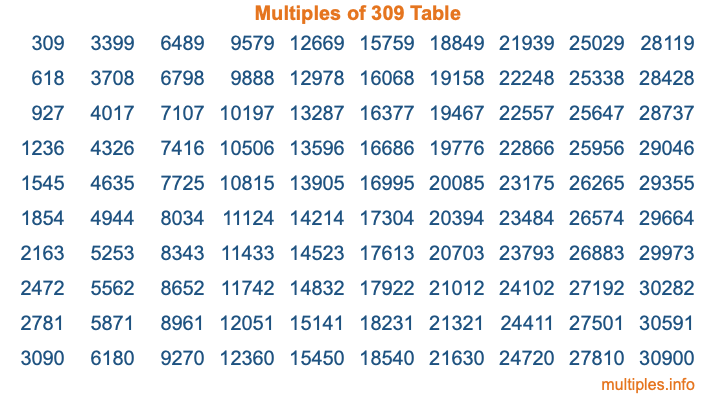Multiples of 309Welcome to the Multiples of 309 page. Here we will first teach you everything you will ever need to know about the multiples of 309, and then give you a study guide summary of everything we taught you to make sure you remember it all. Use this page to look up facts and learn information about the multiples of 309. This page will make you a multiples of three hundred nine expert!

Definition of Multiples of 309
Multiples of 309 are all the numbers that when divided by 309 equal an integer. Each of the multiples of 309 are called a multiple. A multiple of 309 is created by multiplying 309 by an integer.

Therefore, to create a list of multiples of 309, you start with 1 multiplied by 309, then 2 multiplied by 309, then 3 multiplied by 309, and so on for as long as you want. Thus, the list of the first five multiples of 309 is 309, 618, 927, 1236, and 1545. To see a larger list of multiples of 309, see the printable image of Multiples of 309 further down on this page. We also have a category where you can choose any nth multiple of 309.

Multiples of 309 Checker
The Multiples of 309 Checker below checks to see if any number of your choice is a multiple of 309. In other words, it checks to see if there is any number (integer) that when multiplied by 309 will equal your number. To do that, we divide your number by 309. If the the quotient is an integer, then your number is a multiple of 309.

Is  a multiple of 309?

Least Common Multiple of 309 and ...
A Least Common Multiple (LCM) is the lowest multiple that two or more numbers have in common. This is also called the smallest common multiple or lowest common multiple and is useful to know when you are adding our subtracting fractions. Enter one or more numbers below (309 is already entered) to find the LCM.

Check out our LCM Calculator if you need more details about the Least Common Multiple or if you need the LCM for different numbers for adding and subtraction fractions.

nth Multiple of 309
As we stated above, 309 is the first multiple of 309, 618 is the second multiple of 309, 927 is the third multiple of 309, and so on. Enter a number below to find the nth multiple of 309.

th multiple of 309

Multiples of 309 vs Factors of 309
309 is a multiple of 309 and a factor of 309, but that is where the similarities end. All postive multiples of 309 are 309 or greater than 309. All positive factors of 309 are 309 or less than 309.

Below is the beginning list of multiples of 309 and the factors of 309 so you can compare:

Multiples of 309: 309, 618, 927, 1236, 1545, etc.

Factors of 309: 1, 3, 103, 309

As you can see, the multiples of 309 are all the numbers that you can divide by 309 to get a whole number. The factors of 309, on the other hand, are all the whole numbers that you can multiply by another whole number to get 309.

It's also interesting to note that if a number (x) is a factor of 309, then 309 will also be a multiple of that number (x).

Multiples of 309 vs Divisors of 309
The divisors of 309 are all the integers that 309 can be divided by evenly. Below is a list of the divisors of 309.

Divisors of 309: 1, 3, 103, 309

The interesting thing to note here is that if you take any multiple of 309 and divide it by a divisor of 309, you will see that the quotient is an integer.

Multiples of 309 Table
Below is an image of the first 100 multiples of 309 in a table. The table is in chronological order, column by column. The first column has the first ten multiples of 309, the second column has the next ten multiples of 309, and so on.The Multiples of 309 Table is also referred to as the 309 Times Table or Times Table of 309. You are welcome to print out our table for your studies.

Negative Multiples of 309
Although not often discussed or needed in math, it is worth mentioning that you can make a list of negative multiples of 309 by multiplying 309 by -1, then by -2, then by -3, and so on, to get the following list of negative multiples of 309:

-309, -618, -927, -1236, -1545, etc.

Multiples of 309 Summary
Below is a summary of important Multiples of 309 facts that we have discussed on this page. To retain the knowledge on this page, we recommend that you read through the summary and explain to yourself or a study partner why they hold true.

There are an infinite number of multiples of 309.

A multiple of 309 divided by 309 will equal a whole number.

309 divided by a factor of 309 equals a divisor of 309.

The nth multiple of 309 is n times 309.

The largest factor of 309 is equal to the first positive multiple of 309.

309 is a multiple of every factor of 309.

309 is a multiple of 309.

A multiple of 309 divided by a divisor of 309 equals an integer.

309 divided by a divisor of 309 equals a factor of 309.

Any integer times 309 will equal a multiple of 309.

Multiples of a Number
Here you can get the multiples of another number, all with the same attention to detail as we did for multiples of 309 on this page.

Multiples of
Multiples of 310
Did you find our page about multiples of three hundred nine educational? Do you want more knowledge? Check out the multiples of the next number on our list!